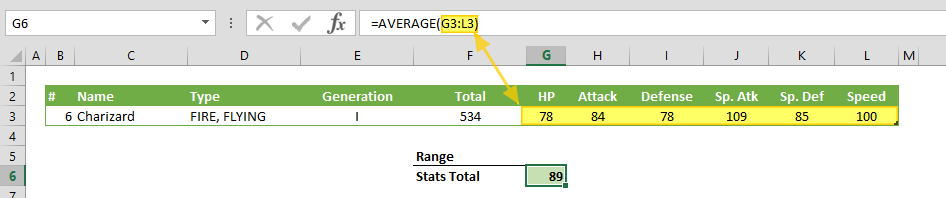The AVERAGE function can calculate the average (arithmetic mean) of the values in a range of cells. In this guide, we’re going to show you how to use the function and also throw in some tips and error handling methods.

• All versions

# Syntax

AVERAGE(number1,[number2],...)

# Arguments

 number1 The first number or numbers you want to calculate. You can put a static number like 4, a cell reference like B6, or a cell range like B2:B8. [number2] Optional. The second number or numbers you want to calculate up to 255 numbers.

Examples

## Example 1

=AVERAGE(78,84,78,109,85,100)
formula simply AVERAGEs all numbers in the arguments.## Example 2

=AVERAGE(G3,H3,I3,J3,K3,L3)
formula AVERAGEs each value in referred cells.## Example 3

=AVERAGE(G3:L3)
formula AVERAGEs values in each cells in referred range. Any valid range can be used with the function.# Tips

• The function ignores the string values. However, please keep in mind that date or time values are serial numbers for Excel, not strings.
• If you want to calculate the average of the values that meet certain criteria, use the AVERAGEIF function or the AVERAGEIFS function.

# Issues

## #####

The column is too narrow for the result of the function. The solution is to increase the width of the column.

## Error

If there is an error in referred cells or ranges,it returns the same error.